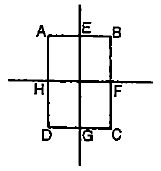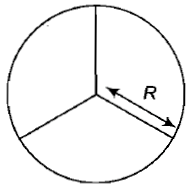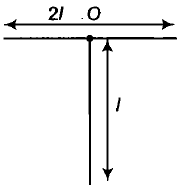Two rings of the same radius and mass are placed such that their centers are at a common point and their planes are perpendicular to each other. The moment of inertia of the system about an axis passing through the center and perpendicular to the plane of one of the rings is (mass of the ring and radius = r)

1.  $\frac{1}{2}{\mathrm{mr}}^{2}$

2.  ${\mathrm{mr}}^{2}$

3.  $\frac{3}{2}{\mathrm{mr}}^{2}$

4.  $2{\mathrm{mr}}^{2}$

Concept Questions :-

Moment of inertia
High Yielding Test Series + Question Bank - NEET 2020

Difficulty Level:

In a rectangle ABCD (BC = 2AB). The moment of inertia along which axis will be minimum?

1.  BC

2.  BD

3.  HF

4.  EGConcept Questions :-

Moment of inertia
High Yielding Test Series + Question Bank - NEET 2020

Difficulty Level:

A wheel comprises of a ring of radius R and mass M and three spokes of mass m each. The moment of inertia of the wheel about its axis is1.

2.

3.

4.

Concept Questions :-

Moment of inertia
High Yielding Test Series + Question Bank - NEET 2020

Difficulty Level:

The angular velocity of a body changes from $\frac{1}{25}$ rev./sec to 1 rev./sec. without applying any torque. The ratio of its radius of gyration in two situations is :

1.  5: 1

2.  1: 5

3.  1: 25

4.  25: 1

Concept Questions :-

Angular momentum
High Yielding Test Series + Question Bank - NEET 2020

Difficulty Level:

A solid cylinder and a solid sphere both having the same mass and radius, are released from a rough inclined plane. Both roll without slipping. Then,

1.  the force of friction that acts on the two is the same

2.  the force of friction is greater in case of a sphere than for a cylinder

3.  the force of friction is greater in case of a cylinder than for a sphere

4.  the force of friction will depend on the nature of the surface of the body that is moving and that of the inclined surface and is independent of the shape and size of the moving body

Concept Questions :-

Rolling motion
High Yielding Test Series + Question Bank - NEET 2020

Difficulty Level:

A homogeneous disc of mass 2 kg and radius 15 cm is rotating about its axis (which is fixed) with an angular velocity of 4 rad/sec. The linear momentum Of the disc is

1.  1.2 kg m/sec

2.  1.0 kg m/sec

3.  0.6 kg m/sec

4.  None of these

Concept Questions :-

Linear momentum
High Yielding Test Series + Question Bank - NEET 2020

Difficulty Level:

A rigid body rotates about a fixed axis with variable angular velocity equal to , at the time t, where $\alpha ,\beta$ are constants. The angle through which it rotates before its stops

1.  $\frac{{\alpha }^{2}}{2\beta }$

2.  $\frac{{\alpha }^{2}-{\beta }^{2}}{2\alpha }$

3.  $\frac{{\alpha }^{2}-{\beta }^{2}}{2\beta }$

4.

Concept Questions :-

Rotation motion : Introduction
High Yielding Test Series + Question Bank - NEET 2020

Difficulty Level:

For the uniform T shaped structure, with mass 3M, the moment of inertia about an axis normal to the plane and passing through O would be1.  $\frac{2}{3}{\mathrm{MI}}^{2}$

2.  ${\mathrm{MI}}^{2}$

3.  $\frac{{\mathrm{MI}}^{2}}{3}$

4.  None of these

Concept Questions :-

Moment of inertia
High Yielding Test Series + Question Bank - NEET 2020

Difficulty Level:

High Yielding Test Series + Question Bank - NEET 2020

Difficulty Level:

An automobile moves on a road with a speed of 54 kmh${}^{-1}$. The radius of its wheel is 0.45 m and moment of inertia of the wheel about its axis of rotation is 3 kg m${}^{2}$. If the vehicle is brought to rest in 15s, the magnitude of average torque transmitted by its brakes to the wheel is

1. 8.58

2. 10.86

3. 2.86

4. 6.66

Concept Questions :-

Torque